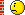# Problems with TMinuit

Hi everyone,

I’m trying to minimize a chi square function, this is the FCN code:

``````void myFCNres( int &npar , double * gin , double &f , double * par , int flag ) {

double chi2 = 0;

for ( int i = 1 ; i <= datasetNHres->GetSize()-2 ; ++i ) {

par = Emin + (Emax-Emin)/(2*N_div) + (i-1)*(Emax-Emin)/N_div;
chi2 += pow( ( integrand->Integral( 1.804 , 8 , par , 1E-15) - datasetNHres->GetBinContent(i)) / datasetNHres->GetBinError(i) ,2);
}

chi2 += pow( (par - p) / sig ,2) +
pow( (par - p) / sig ,2) +
pow( (par - p) / sig ,2) +
pow( (par + p) / sig ,2);

f = chi2;
}``````

The result of running is that none of the parameters has changed, just like my function does not depend on any of them. Do you see problems with FCN logic or I have to look for the bug elsewhere?

You find the complete minimization code in the attachments with the datafile if you want to run it. You can use it directly as a macro with the standard

``.x minuitFitRes_local.cc``

command.

Thanks,
Luigi
minuitFitRes_local.cc (6.94 KB)
datasetRes.root (6.95 KB)

Please HELP me if you can, I’m still stuck.Everything could be helpful, also general impressions or other suggestions e.g. different approaches to the general problem. Many thanks.

``root  .x minuitFitRes_local.cc++``

minuitFitRes_local.cc (7.57 KB)

Thanks!

I suppose the problem was not retrieving parameter values in the FCN code after every iteration, am I right? If yes, why? I’ve written a very similar program (without that integral in the chisquare function) and it works fine also without those lines.